# Matrix syntax in CTL

Something that sometimes confuses people when trying to read a color transform expressed in CTL and/or translate it to another lanugage is the way that matrices are expressed in CTL.

In CTL, the order of operands in vector-times-matrix functions is swapped from “textbook” convention. Textbooks often use “matrix-times-column vector” notation, but CTL functions expect “row vector-times-matrix” syntax. (See page 40-41 of CtlManual.pdf for more information )

To convert textbook notation to row vector-times-matrix notation requires transposing all matrices and swapping the left and right operands of multiplications.

### Textbook notation

Textbooks write the operation of a matrix times a vector as: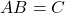where

•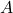is a ‘3 row by 3 column’ matrix (i.e. 3×3)
•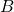is a ‘3 row by 1 column’ vector (i.e. 3×1)
•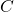is a ‘3 row by 1 column’ vector (i.e. 3×1)

With lowercase letter variables substituted as, and with the context of RGB color vectors substituted forand, this becomes: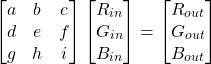which, when written out fully is equivalent to: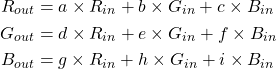### CTL notation

In CTL, the same operation would be:

const float A =                 // This is a 3 row by 3 column vector (3x3)
{                                     // * Note that it is transposed from
{ a,  d,  g},                       //   the textbook representation.
{ b,  e,  h},
{ c,  f,  i}
};

float B = { R_in, G_in, B_in };    // This is a 1 row by 3 column vector (1x3)

float C = mult_f3_f33( B, A);      // Results in a 1 row by 3 column vector (1x3)

float R_out = C;
float G_out = C;
float B_out = C;

### Values for ACES Transforms

In the ACES transforms, built-in CTL functions are used for vector/matrix operations.

For absolute clarity of the math being performed, the an example matrix as it appears in the ACES reference CTL transforms is listed below, followed by the explicit math that would calculate the same result as the built-in functions when using that matrix as the operator.

Note that the values of the matrix coefficients are rounded to 10 decimal places, but in actual implementations, indexing into stored variables at full precision of the specific implementation is preferred.

#### AP0-to-XYZ Matrix

In the code, matrix is created by:

const float AP0_2_XYZ_MAT = RGBtoXYZ( AP0, 1.0);


and the operator would be:

const float RGB_out = mult_f3_f44( RGB_in, AP0_2_XYZ_MAT);


Equivalent math, written out explicitly:

R_out =  0.9525523959 * R_in +  0.0000000000 * G_in +  0.0000936786 * B_in;
G_out =  0.3439664498 * R_in +  0.7281660966 * G_in + -0.0721325464 * B_in;
B_out =  0.0000000000 * R_in +  0.0000000000 * G_in +  1.0088251844 * B_in;